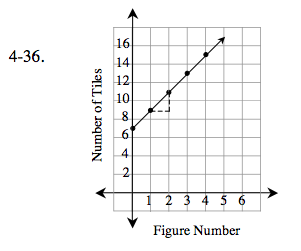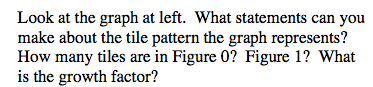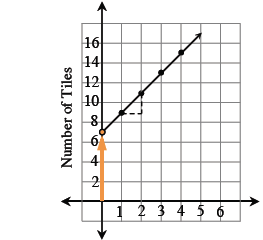### Home > CAAC > Chapter 4 > Lesson 4.1.4 > Problem4-36

4-36.See Graph.Use the same method used to find the number of tiles in Figure 1.

How is the number of tiles changing?

$\frac{\text{change in }y}{\text{change in }x} = \frac{2}{1}$

growth triangle = 2

Find an equation in the form of y = mx + b.